# Noise Parameter Extraction using Source Pull

New and improved for March 2012, thanks to Maury Microwave!

Source pull involves varying the source impedance presented to a device under test and monitoring a single or set of performance parameters.  When used in conjunction with noise figure analyzer (NFA), source pull is often used to measure noise figure (or noise power) as a function of source impedance presented to the DUT.

The first question is “what is noise figure?”  Noise figure is a value which explains the level of noise produced when a signal passes through an active device.  Noise causes degradation in the original signal, to the extent that the content within the original signal might be unrecoverable. The lower the noise figure, the cleaner the resulting signal (i.e. a NF of 0.3dB is lower and more advantageous to a NF of 1dB or 5dB).  Amplifiers have associated noise figures normally listed in their data sheets.

The second question is 'why do we need to measure noise figure under non-50 ohm conditions?”  The term noise figure normally refers to the 50 ohm noise figure of an amplifier which is at or near the minimum possible noise figure for that amplifier it was designed well.  However, in the case of unmatched transistors, the minimum noise figure will not likely occur at 50 ohm but at some other impedance.  It is therefore critical for low noise amplifier designers to understand how to best match their transistor to obtain the least amount of noise possible.  The value of the least amount of noise is referred to as the minimum noise figure, or Fmin.  "NFmin" usually refers to the decibel value of "Fmin" which is a linear ratio. This minimum noise figure occurs at some specific impedance referred to as Gamma,opt in real and imaginary values.  A fourth value, noise resistance or Rn, represents the rate of change of the level of noise when varying the source impedance presented to the DUT. Lower Rn means a more desirable, shallower slope where noise figure does not rapidly increase as impedance moves away from Gamma,opt.  The four noise parameters (Fmin, Gopt, Rn) are graphically represented by the image shown below. Collapsed onto the two dimensions of a Smith chart, the contours of constant noise figure (in linear or decibel format) form perfect circles.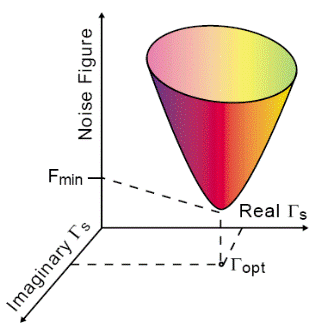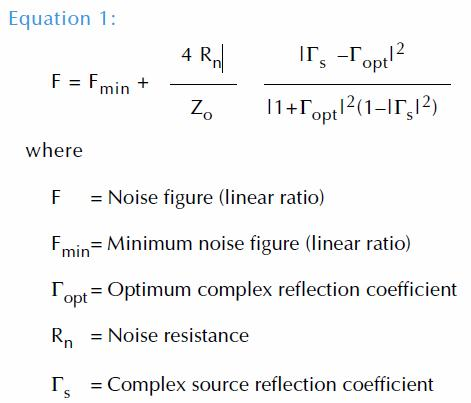The noise figure at any impedance can be described by Equation 1.  Since there are four unknown noise parameters, it is theoretically possible to use only four measurements to solve the simultaneous equations.  However, it is better to measure an overdetermined set of results and use least-mean-square technique to extract the four parameters.

A second technique, using noise power instead of noise figure, can be used to solve for the four noise parameters.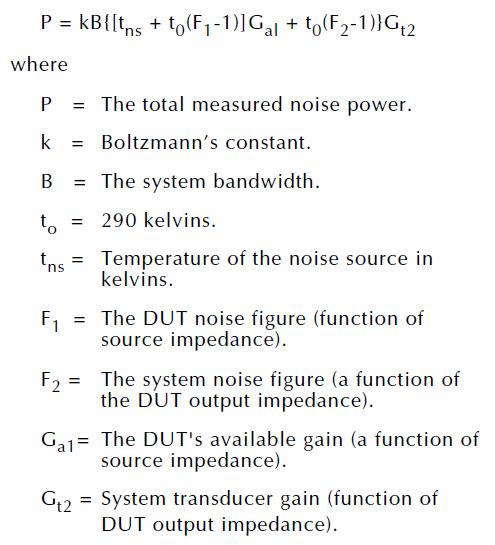A typical noise parameter measurement system, used to determine the four noise parameters, consists of a vector network analyzer (VNA) to measure S-Parameters (such as a Keysight PNA, or PNA-X), a noise figure analyzer (NFA) to measure noise power (such as an Keysight N8975A, or integrated 029 option within the PNA-X), a source tuner to vary the impedance presented to the DUT (such as Maury Microwave MT98x-series tuners), a noise source to present hot and cold states to the DUT, and miscellaneous components such as switches, cables, adapters… as well as noise parameter measurement software (such as Maury Microwave ATS software).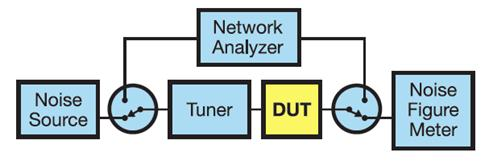A 2008 paper presented at the 72nd ARFTG conference by Gary Simpson of Maury Microwave Corporation, David Ballo and Joel Dunsmore of Agilent Technologies (now Keysight), revolutionized the way noise parameters are measured.  Instead of calibrating the noise parameter system and measuring the DUT on a frequency-by-frequency basis, a faster, more accurate method was developed that improved the overall calibration and measurement time by a factor of 100X-400X, bringing measurements that could once take tens or hundreds of hours to tens of minutes.

The new method relied on two key features that contributed to the breakthrough speed improvement: 1) the tuner is characterized with one set of states (physical tuner positions) that are selected to give a reasonable impedance spread over the frequency band of interest; and 2) the noise power measurement is swept over the frequency range at each state, so that the tuner only moves to each position once. This takes advantage of the fast sweep capability of modern instruments, as well as saving time by minimizing tuner movement.

Speed improvements were presented as part of the ARFTG paper.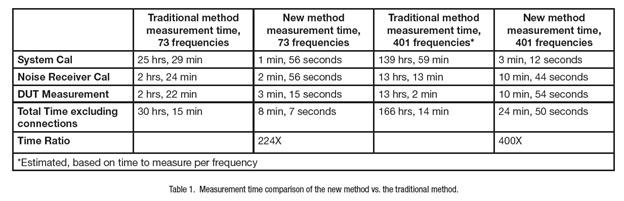Accuracy improvements were also presented as part of the ARFTG paper, as shown in the two plots below: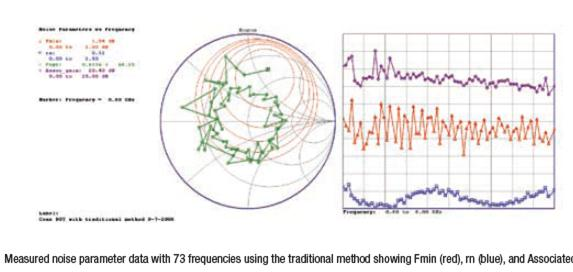Unimproved accuracy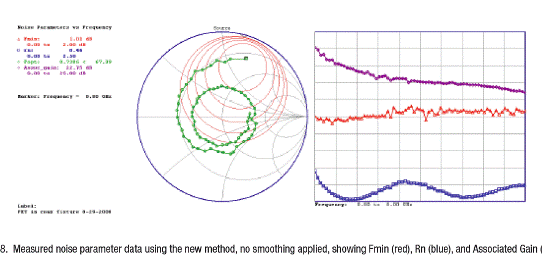Improved accuracy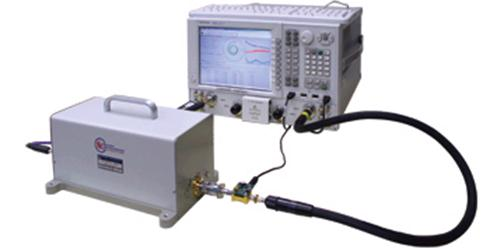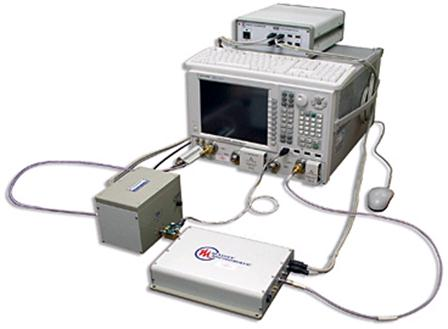Author : Unknown Editor